# Slab + interval stats and geoms

## Introduction

This vignette describes the slab+interval geoms and stats in `ggdist`. This is a flexible family of stats and geoms designed to make plotting distributions (such as priors and posteriors in Bayesian models, or even sampling distributions from other models) straightforward, and support a range of useful plots, including intervals, eye plots (densities + intervals), CCDF bar plots (complementary cumulative distribution functions + intervals), gradient plots, and histograms.

## Setup

The following libraries are required to run this vignette:

``````library(dplyr)
library(tidyr)
library(distributional)
library(ggdist)
library(ggplot2)
library(cowplot)
library(patchwork)

theme_set(theme_ggdist())``````

## Roadmap: It all starts with “slabinterval”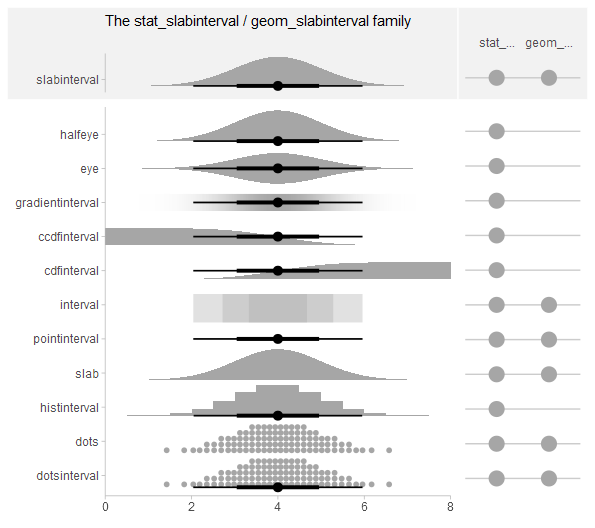`ggdist` has a pantheon of geoms and stats that stem from a common root: `geom_slabinterval()` and `stat_slabinterval()`. These geoms consist of a “slab” (say, a density or a CDF), one or more intervals, and a point summary. These components may be computed in a number of different ways, and different variants of the geom will or will not include all components.

The base `geom_slabinterval()` uses a variety of custom aesthetics to create the composite geometry: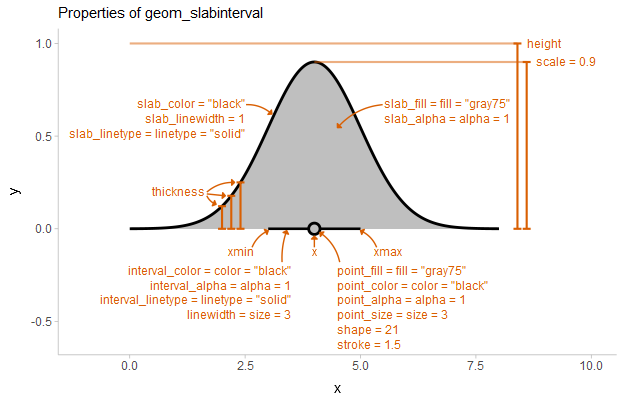Depending on whether you want a horizontal or vertical orientation, you can provide `ymin` and `ymax` instead of `xmin` and `xmax`. By default, some aesthetics (e.g., `fill`, `color`, `size`, `alpha`) set properties of multiple sub-geometries at once. For example, the `color` aesthetic by default sets both the color of the point and the interval, but can also be overridden by `point_color` or `interval_color` to set the color of each sub-geometry separately.

`geom_slabinterval()` is most useful when paired with `stat_slabinterval()`, which will automatically calculate intervals, densities, and cumulative distribution functions, and maps these onto endpoints of the interval sub-geometry or the `thickness` of the slab sub-geometry.

Using `geom_slabinterval()` and `stat_slabinterval()` directly is not always advisable: they are highly configurable on their own, but this configurability requires remembering a number of combinations of options to use. For quick plotting, ggdist contains a number of pre-configured, easier-to-remember shortcut stats and geoms built on top of the slabinterval:

• Shortcut geoms, starting with `geom_`, are meant to be used on already-summarized data: `geom_pointinterval()` and `geom_interval()` (for data summarized into intervals) and `geom_slab()` (for data summarized into function values, like densities or cumulative distribution functions).

• Shortcut stats, starting with `stat_`, which compute relevant summaries (densities, CDFs, points, and/or intervals) before forwarding the summaries to their geom. Some have geom counterparts (e.g. `stat_interval()` corresponds to `geom_interval()`, except the former applies to sample data and the latter to already-summarized data). Many of these stats do not currently have geom counterparts (e.g. `stat_ccdfinterval()`), as they are primarily differentiated based on what kind of statistical summary they compute. If you’ve already computed a function (such as a density or CDF), you can just use `geom_slabinterval()` directly. These stats can be used on two types of data, depending on what aesthetic mappings you provide:

• Sample data; e.g. draws from a data distribution, bootstrap distribution, Bayesian posterior distribution (or any other distribution, really). To use the stats on sample data, map sample values onto the `x` or `y` aesthetic.

• Distribution objects and analytical distributions. To use the stats on this type of data, you must use the `xdist`, or `ydist` aesthetics, which take distributional objects, `posterior::rvar()` objects, or distribution names (e.g. `"norm"`, which refers to the Normal distribution provided by the `dnorm/pnorm/qnorm` functions).

All slabinterval geoms can be plotted horizontally or vertically. Depending on how aesthetics are mapped, they will attempt to automatically determine the orientation; if this does not produce the correct result, the orientation can be overridden by setting `orientation = "horizontal"` or `orientation = "vertical"`.

We’ll start with one of the most common existing use cases for these kinds geoms: eye plots.

## Eye plots and half-eye plots

### On sample data

Eye plots combine densities (as violins) with intervals to give a more detailed picture of uncertainty than is available just by looking at intervals.

For these first few demos we’ll use these data:

``````set.seed(1234)
df = tribble(
~group, ~subgroup, ~value,
"a",          "h", rnorm(1000, mean = 5),
"b",          "h", rnorm(1000, mean = 7, sd = 1.5),
"c",          "h", rnorm(1000, mean = 8),
"c",          "i", rnorm(1000, mean = 9),
"c",          "j", rnorm(1000, mean = 7)
) %>%
unnest(value)``````

We can summarize it at the group level using an eye plot with `stat_eye()` (ignoring subgroups for now):

``````df %>%
ggplot(aes(y = group, x = value)) +
stat_eye() +
ggtitle("stat_eye()")``````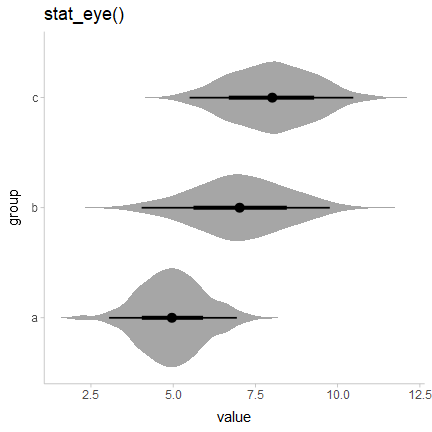Users of older versions of `tidybayes` (which used to contain the `ggdist` geoms) might have used `geom_eye()`, which is the older spelling of `stat_eye()`. Due to the name standardization in version 2 of `tidybayes` (see the description above), `stat_eye()` is now the preferred spelling. `geom_eye()` will continue to work for now, but is deprecated and may throw a warning in future versions.

We can also use `stat_halfeye()` instead to get densities instead of violins:

``````df %>%
ggplot(aes(y = group, x = value)) +
stat_halfeye() +
ggtitle("stat_halfeye()")``````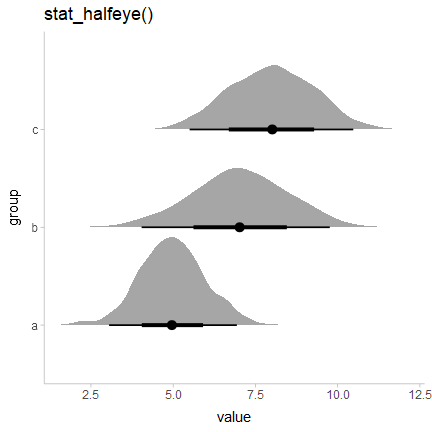Or use the `side` parameter to more finely control where the slab (in this case, the density) is drawn:

``````p = df %>%
ggplot(aes(x = group, y = value)) +
panel_border()

plot_grid(ncol = 3, align = "hv",
p + stat_eye(side = "left") + labs(title = "stat_eye()", subtitle = "side = 'left'"),
p + stat_eye(side = "both") + labs(subtitle = "side = 'both'"),
p + stat_eye(side = "right")  + labs(subtitle = "side = 'right'")
)``````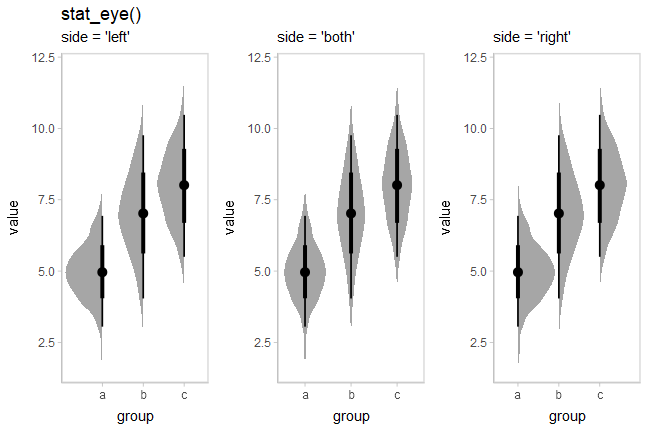Note how the above chart was drawn vertically instead of horizontally: all slabinterval geoms automatically detect their orientation based on the input data. For example, if you use a factor on one axis (say the `x` axis below), the geom will be drawn along the other axis:

``````df %>%
ggplot(aes(x = group, y = value)) +
stat_halfeye() +
ggtitle("stat_halfeye()")``````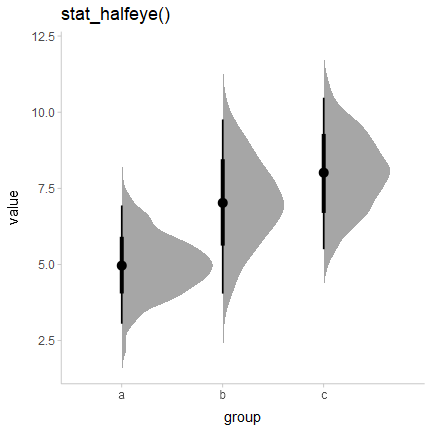If automatic detection of the desired axis fails, you can specify it manually; e.g. with `stat_halfeye(orientation = 'vertical')` or `stat_halfeye(orientation = 'horizontal')`.

The `side` parameter works for horizontal geoms as well. `"top"` and `"right"` are considered synonyms, as are `"bottom"` and `"left"`; either form works with both horizontal and vertical versions of the geoms:

``````p = df %>%
ggplot(aes(x = value, y = group)) +
panel_border()

plot_grid(ncol = 3, align = "hv",
# side = "left" would give the same result
p + stat_eye(side = "left") + ggtitle("stat_eye()") + labs(subtitle = "side = 'bottom'"),
p + stat_eye(side = "both") + labs(subtitle = "side = 'both'"),
# side = "right" would give the same result
p + stat_eye(side = "right") + labs(subtitle = "side = 'top'")
)``````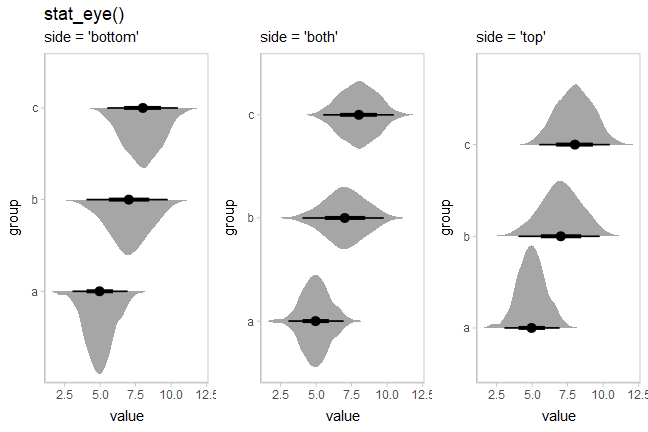Eye plots are also designed to support dodging through the standard mechanism of `position = "dodge"`. Unlike with geom_violin(), densities in groups that are not dodged (here, ‘a’ and ‘b’) have the same area and max width as those in groups that are dodged (‘c’):

``````df %>%
ggplot(aes(x = group, y = value, fill = subgroup)) +
stat_eye(position = "dodge") +
ggtitle("stat_eye(position = 'dodge')")``````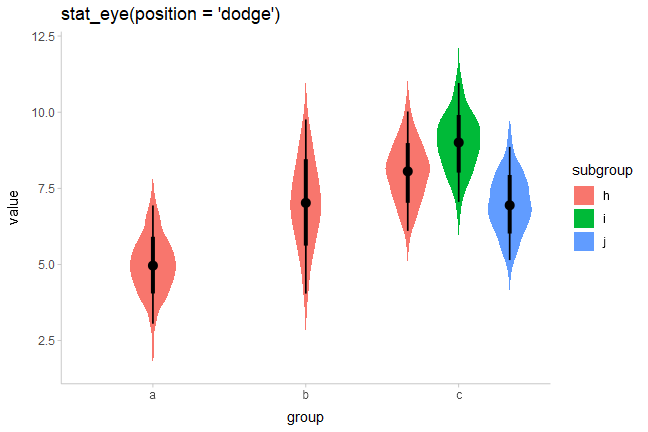Dodging works whether geoms are horizontal or vertical.

### On analytical distributions

The same set of (half-)eye plot stats designed for sample data described above can be used on analytical distributions or distribution vectors by using the `xdist`/`ydist` aesthetics instead of `x`/`y`. These stats accept specifications for distributions in one of two ways:

Using distribution names as character vectors: this format uses aesthetics as follows:

• `xdist`, `ydist`, or `dist`: the name of the distribution, following R’s naming scheme. This is a string which should have `"p"`, `"q"`, and `"d"` functions defined for it: e.g., “norm” is a valid distribution name because the `pnorm()`, `qnorm()`, and `dnorm()` functions define the CDF, quantile function, and density function of the Normal distribution.
• `args` or `arg1`, … `arg9`: arguments for the distribution. If you use `args`, it should be a list column where each element is a list containing arguments for the distribution functions; alternatively, you can pass the arguments directly using `arg1`, … `arg9`.

Using distribution vectors from the distributional package or `posterior::rvar()` objects: this format uses aesthetics as follows:

• `xdist`, `ydist`, or `dist`: a distribution vector or `posterior::rvar()` produced by functions such as `distributional::dist_normal()`, `distributional::dist_beta()`, `posterior::rvar_rng()`, etc.

For example, here are a variety of normal distributions describing the same data from the previous example:

``````dist_df = tribble(
~group, ~subgroup, ~mean, ~sd,
"a",          "h",     5,   1,
"b",          "h",     7,   1.5,
"c",          "h",     8,   1,
"c",          "i",     9,   1,
"c",          "j",     7,   1
)``````

We can visualize these distributions directly using `stat_eye()` and the character vector input style to the `ydist`, `arg1`, and `arg2` aesthetics:

``````dist_df %>%
ggplot(aes(x = group, ydist = "norm", arg1 = mean, arg2 = sd, fill = subgroup)) +
stat_eye(position = "dodge") +
ggtitle("stat_eye(position = 'dodge')", 'aes(ydist = "norm", arg1 = mean, arg2 = sd)')``````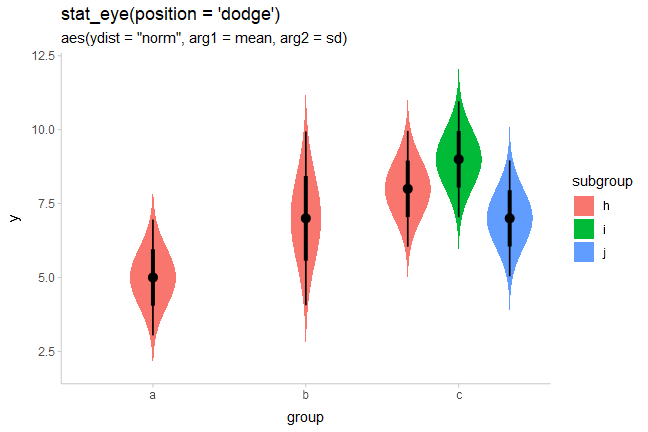Or we can use the `distributional::dist_normal()` function to construct a vector of normal distributions. This syntax is often more compact and expressive than the character-vector format above:

``````dist_df %>%
ggplot(aes(x = group, ydist = dist_normal(mean, sd), fill = subgroup)) +
stat_eye(position = "dodge") +
ggtitle("stat_eye(position = 'dodge')", "aes(ydist = dist_normal(mean, sd))")``````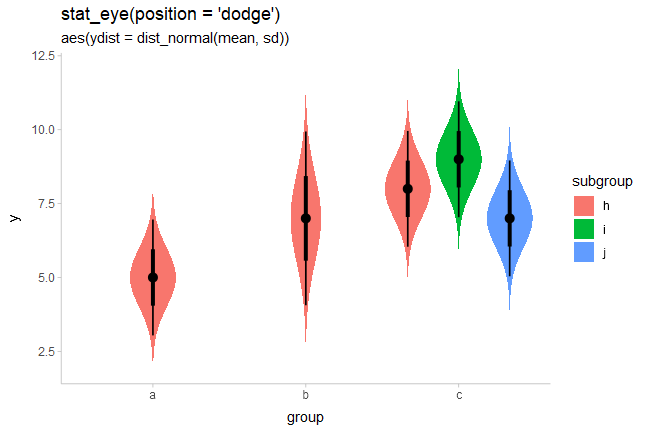This makes it easy to visualize a variety of distributions. E.g., here are some Beta distributions:

``````data.frame(alpha = seq(5, 100, length.out = 10)) %>%
ggplot(aes(y = alpha, xdist = dist_beta(alpha, 10))) +
stat_halfeye() +
labs(
title = "stat_halfeye()",
subtitle = "aes(xdist = dist_beta(alpha, 10), y = alpha)",
x = "Beta(alpha,10) distribution"
)``````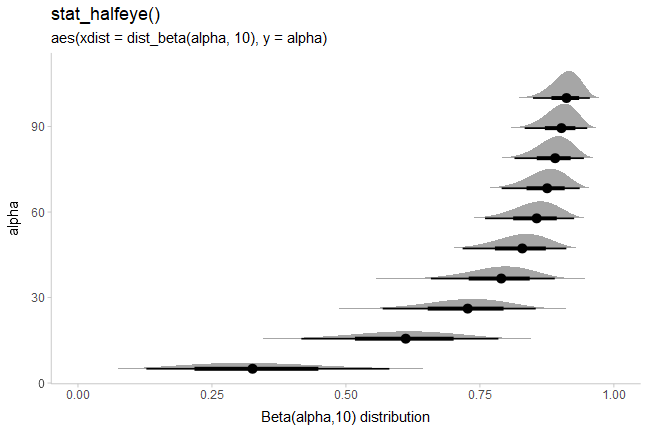If you want to plot all of these on top of each other (instead of stacked), you could turn off plotting of the interval to make the plot easier to read using `stat_halfeye(show_interval = FALSE, ...)`. A shortcut for `stat_halfeye(show_interval = FALSE, ...)` is `stat_slab()`. We’ll also turn off the fill color with `fill = NA` to make the stacking easier to see, and use outline `color` to show the value of `alpha`:

``````data.frame(alpha = seq(5, 100, length.out = 10)) %>%
ggplot(aes(xdist = dist_beta(alpha, 10), color = alpha)) +
stat_slab(fill = NA) +
coord_cartesian(expand = FALSE) +
scale_color_viridis_c() +
labs(
title = "stat_slab()",
subtitle = "aes(xdist = dist_beta(alpha, 10), color = alpha)",
x = "Beta(alpha,10) distribution",
y = NULL
)``````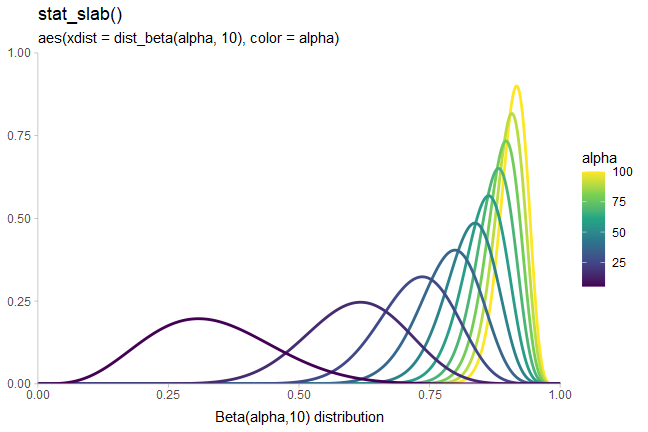The approach of using `arg1`, … `arg9` can work well when comparing similar distributions, but is harder to use with different distribution types. For example, if we wished to compare a Student’s t distribution and a Normal distribution, the arguments may not line up. This is a good case to use list columns and the `args` aesthetic. `ggdist` includes an implementation of the scaled and shifted Student’s t distribution (`dstudent_t()`, `pstudent_t()`, etc) as it is often needed for visualizing frequentist confidence distributions (see `vignette("freq-uncertainty-vis")`) and Bayesian priors:

``````tribble(
~ dist,      ~ args,
"norm",      list(0, 1),
"student_t", list(3, 0, 1)
) %>%
ggplot(aes(y = dist, xdist = dist, args = args)) +
stat_halfeye() +
ggtitle("stat_halfeye()", "aes(xdist = dist, args = args)")``````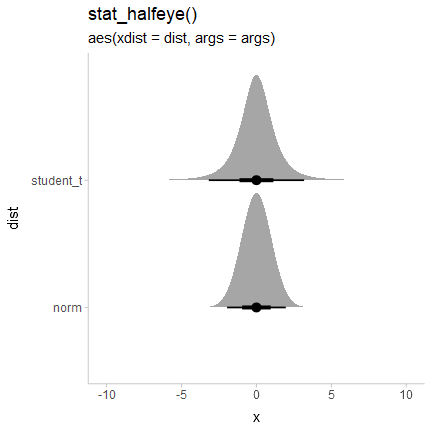### Visualizing priors

A particularly good use of the `xdist`/`ydist` aesthetics is to visualize priors. For example, with `brms` you can specify priors using the `brms::prior()` function, which creates data frames with a `"prior"` column indicating the name of the prior distribution as a string. E.g., one might set some priors on the betas and the standard deviation in a model with something like this:

``````# NB these priors are made up!
priors = c(
prior(normal(0,1), class = b),
prior(lognormal(0,1), class = sigma)
)
priors``````
prior class coef group resp dpar nlpar bound
normal(0, 1) b
lognormal(0, 1) sigma

The `parse_dist()` function can make it easier to visualize these: it takes in string specifications like those produced by `brms``"normal(0,1)"` and `"lognormal(0,1)"` above — and translates them into `.dist` and `.args` columns:

``````priors %>%
parse_dist(prior)``````
prior class coef group resp dpar nlpar bound .dist .args
normal(0, 1) b norm 0, 1
lognormal(0, 1) sigma lnorm 0, 1

Notice that it also automatically translates some common distribution names (e.g. “normal” and “lognormal”) into their equivalent R function names (`"norm"` and `"lnorm"`). This makes it easy to use them with the `xdist`/`ydist` aesthetics in ggdist:

``````priors %>%
parse_dist(prior) %>%
ggplot(aes(y = class, xdist = .dist, args = .args)) +
stat_halfeye() +
labs(
title = "stat_halfeye()",
subtitle = "with brms::prior() and ggdist::parse_dist() to visualize priors",
x = NULL
)``````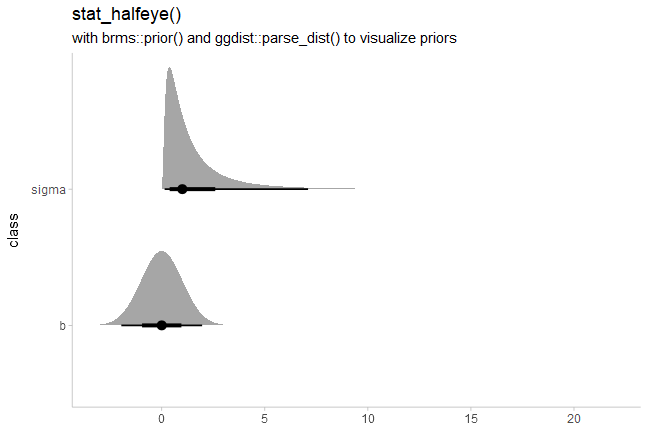### Sharing thickness scaling across geometries

In some cases, such as visualizing priors and posteriors, it can be helpful to use multiple geometries (e.g. one for prior and one for posterior). By default, normalization/scaling of slab thicknesses is controlled by geometries, not by a scale function. This allows various functionality not otherwise possible, such as (1) allowing different geometries to have different thickness scales and (2) allowing the user to control at what level of aggregation (panels, groups, the entire plot, etc) thickness scaling is done via the `normalize` parameter to [geom_slabinterval()].

To override this default behavior and make separate geometries use a shared thickness scale, add `scale_thickness_shared()` to the plot. The difference is illustrated below:

``````prior_post = data.frame(
prior = dist_normal(0, 1),
posterior = dist_normal(0.1, 0.5)
)

separate_scale_plot = prior_post %>%
ggplot() +
stat_halfeye(aes(xdist = posterior)) +
stat_slab(aes(xdist = prior), fill = NA, color = "red") +
labs(
title = "prior (slab) + posterior (halfeye)",
subtitle = "default: no shared thickness scale"
)

shared_scale_plot = prior_post %>%
ggplot() +
stat_halfeye(aes(xdist = posterior)) +
stat_slab(aes(xdist = prior), fill = NA, color = "#e41a1c") +
scale_thickness_shared() +
labs(subtitle = "with scale_thickness_shared()")

separate_scale_plot + shared_scale_plot``````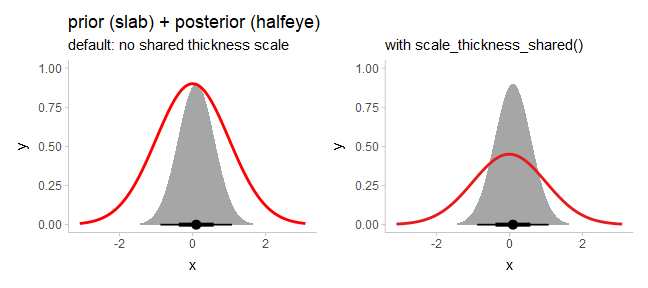With `scale_thickness_shared()` applied, both densities have the same area under their curves.

### Scale transformations of densities

The `stat_slabinterval()` family also adjusts densities appropriately when scale transformations are applied. For example, here is a log-Normal distribution plotted on a log scale:

``````data.frame(dist = "lnorm", logmean = log(10), logsd = 2*log(10)) %>%
ggplot(aes(xdist = dist, arg1 = logmean, arg2 = logsd)) +
stat_halfeye() +
scale_x_log10(breaks = 10^seq(-5,7, by = 2))``````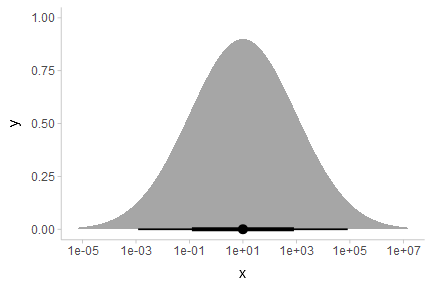As expected, a log-Normal density plotted on the log scale appears Normal. The Jacobian for the scale transformation is applied to the density so that the correct density is shown on the log scale. Internally, ggdist attempts to do symbolic differentiation on scale transformation functions (and if that fails, uses numerical differentiation) to calculate the Jacobian so that the `stat_slabinterval()` family works generically across the different scale transformations supported by ggplot.

### Summing up eye plots: `stat_[half]eye`

All of the stats in this section follow the naming scheme `stat_[half]eye`, where adding `half` to the name to yields half-eyes (density plots) instead of eyes (violins).

Like the remaining shortcut stats, these stats also follow these conventions:

• Map sample values to `x` or `y` to use the stats on sample data.
• Use the `xdist`, `ydist`, and `args` aesthetics for analytical distributions or distributions contained in vector objects, such as distributional or `posterior::rvar()` objects.

## Histogram + interval plots

In some cases you might prefer histograms to density plots. `stat_histinterval()` provides an alternative to `stat_halfeye()` that uses histograms instead of densities; it is roughly equivalent to `stat_slabinterval(slab_type = "histogram")`:

``````p = df %>%
ggplot(aes(x = group, y = value)) +
panel_border()

ph = df %>%
ggplot(aes(y = group, x = value)) +
panel_border()

plot_grid(ncol = 2, align = "hv",
p + stat_histinterval() + labs(title = "stat_histinterval()", subtitle = "horizontal"),
ph + stat_histinterval() + labs(subtitle = "vertical")
)``````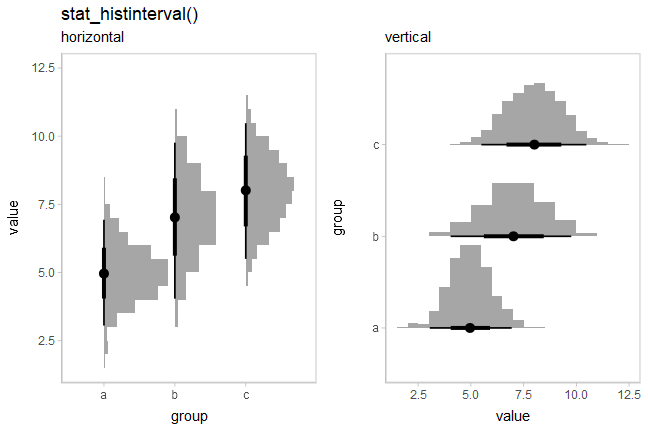You can use the `slab_color` aesthetic to show the outline of the bars. By default the outlines are only drawn along the tops of the bars, as typical tasks with histograms involve area estimation, so the outlines between bars are not strictly necessary and may be distracting. However, if you wish to include those outlines, you can set `outline_bars = TRUE`:

``````plot_grid(ncol = 2, align = "hv",
ph + stat_histinterval(slab_color = "gray45", outline_bars = FALSE) +
labs(title = "stat_histinterval", subtitle = "outline_bars = FALSE (default)"),
ph + stat_histinterval(slab_color = "gray45", outline_bars = TRUE) +
labs(subtitle = "outline_bars = TRUE")
)``````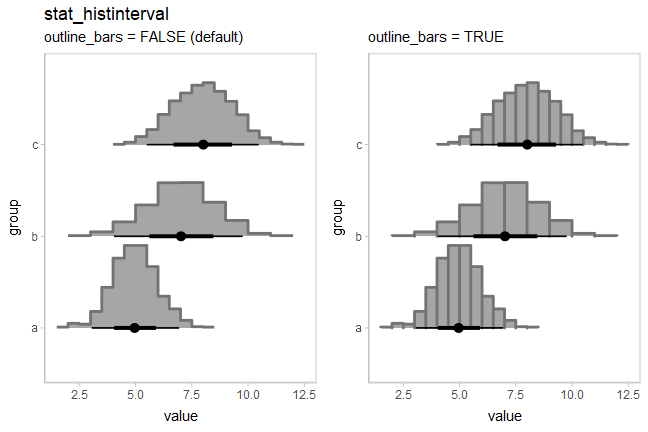### Histograms of analytical distributions

While `stat_histinterval()` will not produce histograms of continuous analytical distributions, the `stat_slabinterval()` family will automatically detect discrete distributions supplied on the `xdist` and `ydist` aesthetics and plot them using stepped histograms instead of densities. As with `stat_histinterval()`, you can choose whether or not to draw outlines between bars of the histogram using `outline_bars = TRUE` or `FALSE` (the default is `FALSE`).

Here is an example of histograms of analytical distributions that also shows a redundant encoding of the density by mapping the `pdf` computed variable onto `fill` (in addition to the default mapping onto `thickness`):

``````tibble(
group = c("a","b","c","d","e"),
lambda = c(13,7,4,3,2)
) %>%
ggplot(aes(x = group)) +
stat_slab(aes(ydist = dist_poisson(lambda), fill = after_stat(pdf))) +
geom_line(aes(y = lambda, group = NA), linewidth = 1) +
geom_point(aes(y = lambda), size = 2.5) +
labs(fill = "Pr(y)") +
ggtitle("stat_slab()", "aes(ydist = dist_poisson(lambda), fill = after_stat(pdf))")``````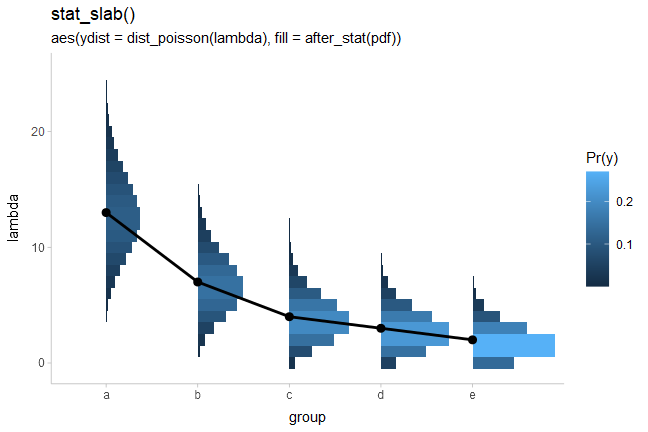This was inspired by an example from Isabella Ghement.

## CCDF bar plots

Another (perhaps sorely underused) technique for visualizing distributions is cumulative distribution functions (CDFs) and complementary CDFs (CCDFs). These can be more effective for some decision-making tasks than densities or intervals, and require fewer assumptions to create from sample data than density plots.

For all of the examples above, both on sample data and analytical distributions, you can replace `[half]eye` with `[c]cdfinterval` to get a stat that creates a CDF or CCDF bar plot.

`stat_ccdfinterval()` is roughly equivalent to `stat_slabinterval(slab_type = "ccdf", justification = 0.5, side = "topleft", normalize = "none", expand = TRUE)`

### On sample data

`stat_[c]cdfinterval` has the following basic combinations:

``````p = df %>%
ggplot(aes(x = group, y = value)) +
panel_border()

ph = df %>%
ggplot(aes(y = group, x = value)) +
panel_border()

plot_grid(ncol = 2, align = "hv",
p + stat_ccdfinterval() + labs(title = "stat_ccdfinterval()", subtitle = "vertical"),
ph + stat_ccdfinterval() + labs(subtitle = "horizontal"),
p + stat_cdfinterval() + labs(title = "stat_cdfinterval()", subtitle = "vertical"),
ph + stat_cdfinterval()  + labs(subtitle = "horizontal")
)``````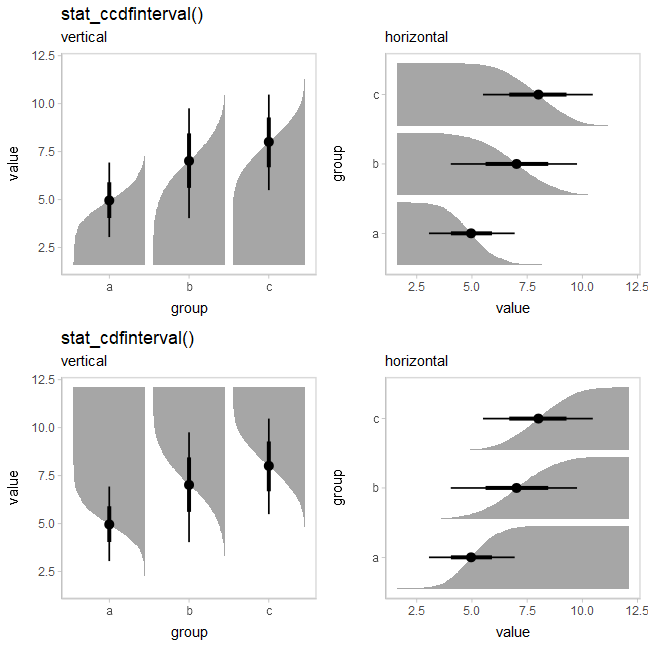The CCDF interval plots are probably more useful than the CDF interval plots in most cases, as the bars typically grow up from the baseline. For example, replacing `stat_eye()` with `stat_ccdfinterval()` in our previous subgroup plot produces CCDF bar plots:

``````df %>%
ggplot(aes(x = group, y = value, fill = subgroup, group = subgroup)) +
stat_ccdfinterval(position = "dodge") +
ggtitle("stat_ccdfinterval(position = 'dodge')") ``````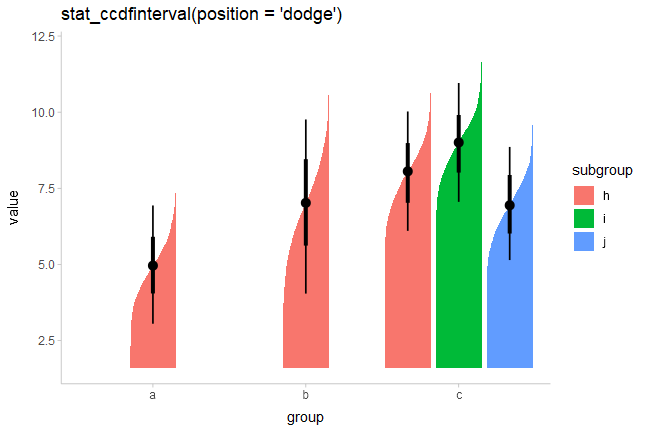The extents of the bars are determined automatically by range of the data in the samples. However, for bar charts it is often good practice to draw the bars from a meaningful reference point (this point is often 0). You can use `ggplot2::expand_limits()` to ensure the bar is drawn down to 0:

``````df %>%
ggplot(aes(x = group, y = value, fill = subgroup)) +
stat_ccdfinterval(position = "dodge") +
expand_limits(y = 0) +
# plus coord_cartesian so there is no space between bars and axis
coord_cartesian(expand = FALSE) +
ggtitle("stat_ccdfinterval(position = 'dodge')")``````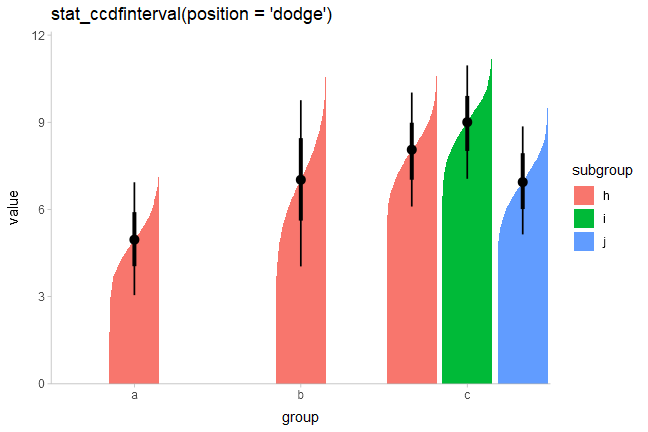You can also adjust the position of the slab relative to the position of the interval using the `justification` parameter:

``````df %>%
ggplot(aes(x = group, y = value, fill = subgroup)) +
stat_ccdfinterval(position = "dodge", justification = 1) +
expand_limits(y = 0) +
coord_cartesian(expand = FALSE) +
ggtitle("stat_ccdfinterval(position = 'dodge', justification = 1)")``````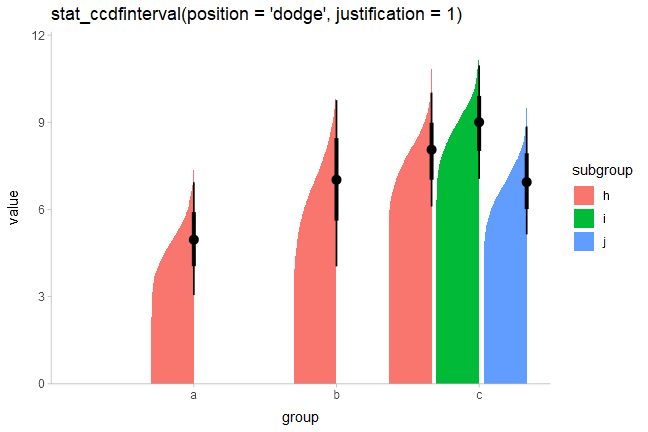The `side` parameter also works in the same way it does with `stat_eye()`. Here we’ll demonstrate it horizontally:

``````p = df %>%
ggplot(aes(x = value, y = group)) +
expand_limits(x = 0) +
panel_border()

plot_grid(ncol = 3, align = "hv",
# side = "left" would give the same result
p + stat_ccdfinterval(side = "bottom") + ggtitle("stat_ccdfinterval()") + labs(subtitle = "side = 'bottom'"),
p + stat_ccdfinterval(side = "both") + labs(subtitle = "side = 'both'"),
# side = "right" would give the same result
p + stat_ccdfinterval(side = "top") + labs(subtitle = "side = 'top'")
)``````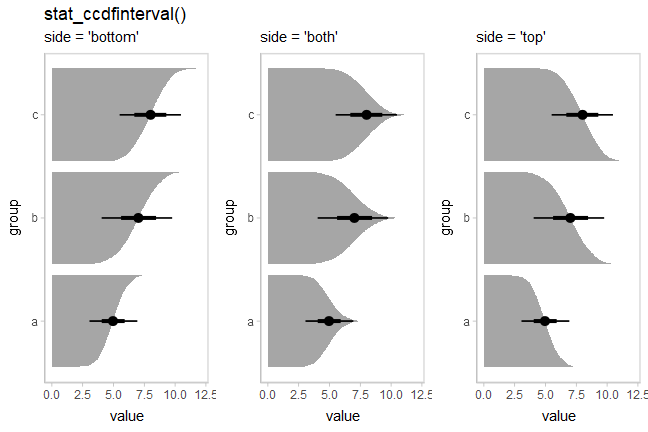### On analytical distributions

You can also use `stat_ccdfinterval()` to visualize analytical distributions or distribution vectors, just as you can with `stat_eye()` and `stat_halfeye()`.

By default, `stat_slabinterval()` uses the quantiles at `p = 0.001` and `p = 0.999` of the distributions to determine their extent (unless the lower or upper limit of the distribution’s support is finite, in which case that value is used). You can change this setting using the `p_limits` parameter, or use `expand_limits()` to ensure a particular value is shown, as before:

``````dist_df %>%
ggplot(aes(x = group, ydist = dist_normal(mean, sd), fill = subgroup)) +
stat_ccdfinterval(position = "dodge") +
expand_limits(y = 0) +
ggtitle(
"stat_ccdfinterval(position = 'dodge')",
"aes(x = dist_normal(mean, sd)) + expand_limits(y = 0)"
) +
coord_cartesian(expand = FALSE)``````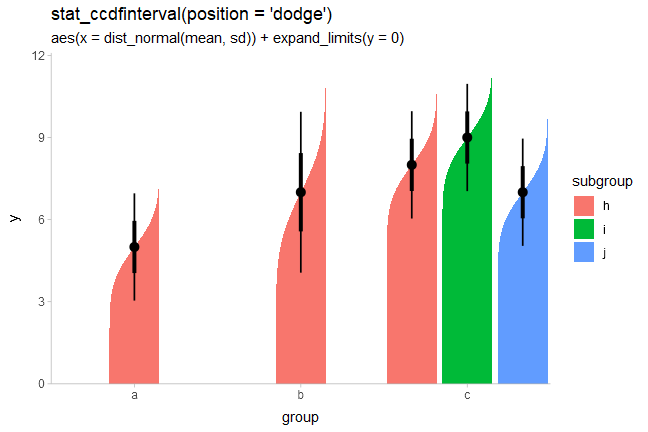### Summing up CDF bar plots

All of the stats in this section follow the naming scheme `stat_[c]cdfinterval`:

• Add `c` to the name to get CCDFs instead of CDFs.
• Use `xdist`/`ydist` instead of `x`/`y` to use the stats on analytical distributions or distribution vectors instead of sample data.
• It can be helpful to use `expand_limits()` to ensure meaningful reference points are included in the plot.

An alternative approach to mapping density onto the `thickness` aesthetic of the slab is to instead map it onto its `alpha` value (i.e., opacity). This is what the `stat_gradientinterval` family does (actually, it uses `slab_alpha`, a variant of the `alpha` aesthetic, described below).

It is roughly equivalent to `stat_slabinterval(aes(slab_alpha = after_stat(f)), thickness = 1, justification = 0.5)`.

### On sample data

For example, replacing `stat_eye()` with `stat_gradientinterval()` produces gradient + interval plots:

``````df %>%
ggplot(aes(x = group, y = value, fill = subgroup)) +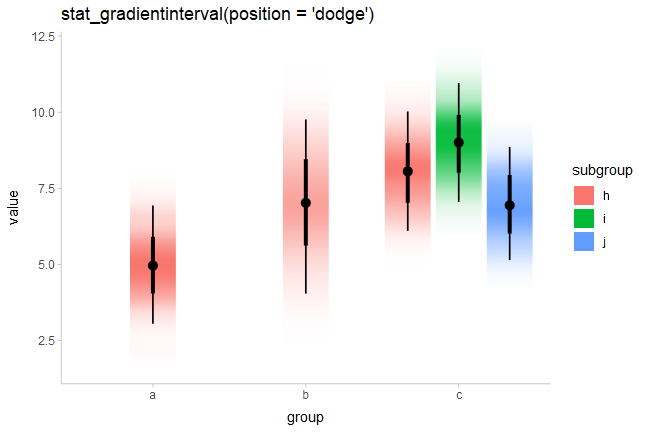`stat_gradientinterval()` maps density onto the `slab_alpha` aesthetic, which is a variant of the ggplot `alpha` scale that specifically targets alpha (opacity) values of the slab portion of `geom_slabinterval()`. This aesthetic has default ranges and limits that are a little different from the base ggplot `alpha` scale and which ensure that densities of 0 are mapped onto opacities of 0. You can use `scale_slab_alpha_continuous()` to adjust this scale’s settings.

Depending on your graphics device, gradients may be “choppy” looking. You can fix this choppiness by setting `fill_type = "gradient"`, which uses a gradient feature introduced in some graphics engines in R 4.1. If you use `stat_gradientinterval()` in R 4.1, you will receive a message suggesting you may want to explicitly set `fill_type = "gradient"` to improve output quality. If you are using R 4.2 or greater, you should not need to set `fill_type = "gradient"` as support for gradients can be auto-detected in that version, but you will get a warning message if you use `stat_gradientinterval()` with a graphics engine that does not support gradients.

``````df %>%
ggplot(aes(x = group, y = value, fill = subgroup)) +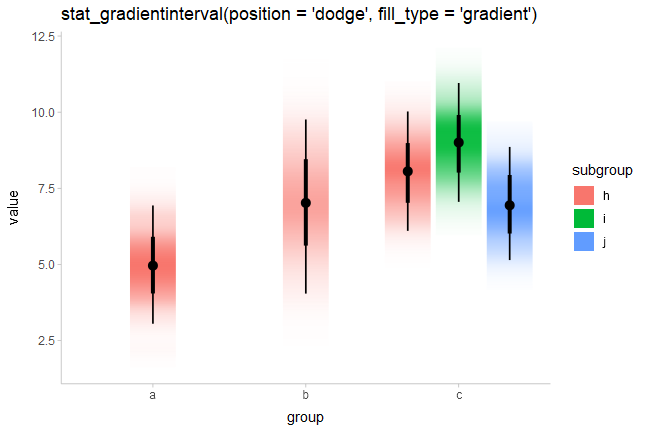As of this writing, in R version 4.1 or greater the graphics devices that support gradients — i.e. device that support the `grid::linearGradient()` function — include `pdf()`, `svg()`, and `png(type = "cairo")`. See here for more about the changes to the R graphics engine.

### On analytical distributions

As with other plot types, you can also use `stat_gradientinterval()` to visualize analytical distributions or distribution vectors:

``````dist_df %>%
ggplot(aes(x = group, ydist = dist_normal(mean, sd), fill = subgroup)) +
labs(
subtitle = "aes(ydist = dist_normal(mean, sd), fill = subgroup)"
)``````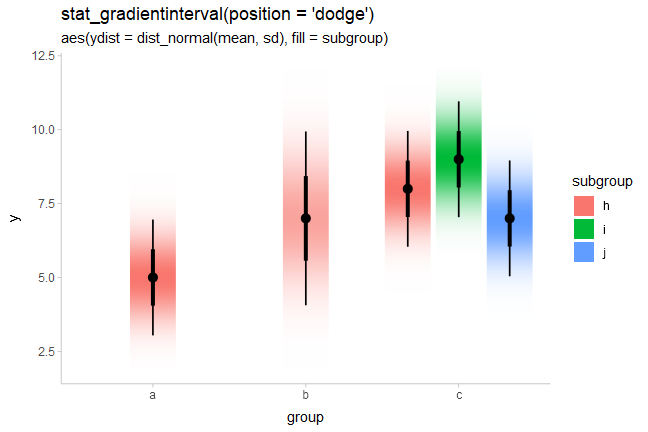## Dotplots

The encodings thus far are continuous probability encodings: they map probabilities or probability densities onto aesthetics like `x`/`y` position or `alpha` transparency. An alternative is discrete or frequency-framing uncertainty visualizations, such as dotplots and quantile dotplots. Dotplots represent distributions by showing each data point, and quantile dotplots extend this idea to analytical distributions by showing quantiles from the distribution as a number of discrete possible outcomes.

### On sample data

For example, replacing `stat_halfeye()` with `stat_dots()` produces dotplots. With so many dots here, the outlines mask the fill, so it makes sense to map `subgroup` to the outline `color` of the dots as well:

``````df %>%
ggplot(aes(x = group, y = value, fill = subgroup, color = subgroup)) +
stat_dots(position = "dodgejust") +
labs(
title = "stat_dots()",
subtitle = "aes(fill = subgroup, color = subgroup))"
)``````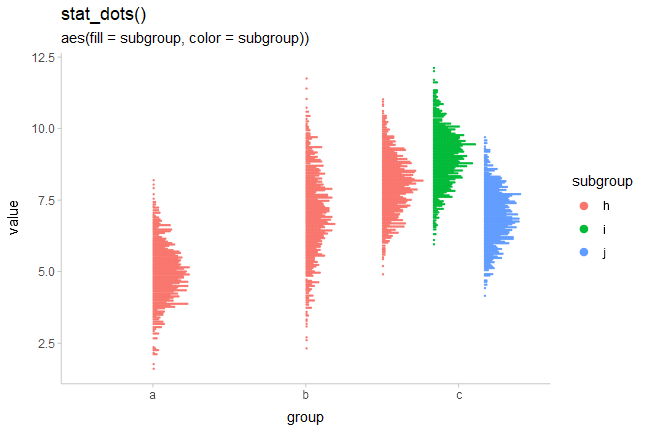Unlike the base `ggplot2::geom_dotplot()` geom, `ggdist::geom_dots()` automatically determines a bin width to ensure that the dot stacks fit within the available space. You can set the `binwidth` parameter manually to override this.

### Quantile dotplots

The above plots are a bit hard to read due to the large number of dots. Particularly when summarizing posterior distributions or predictive distributions, which may have thousands of data points, it can make sense to plot a smaller number of dots (say 20, 50 or 100) that are representative of the full sample. One such approach is to plot quantiles, thereby creating quantile dotplots, which can help people make better decisions under uncertainty (Kay 2016, Fernandes 2018).

The `quantiles` argument to `stat_dots` constructs a quantile dotplot with the specified number of quantiles. Here is one with 50 quantiles, so each dot represents approximately a 2% (1/50) chance. We’ll turn off outline color too (`color = NA`):

``````df %>%
ggplot(aes(x = group, y = value, fill = subgroup)) +
stat_dots(position = "dodgejust", quantiles = 50, color = NA) +
labs(title = "stat_dots(quantiles = 50)")``````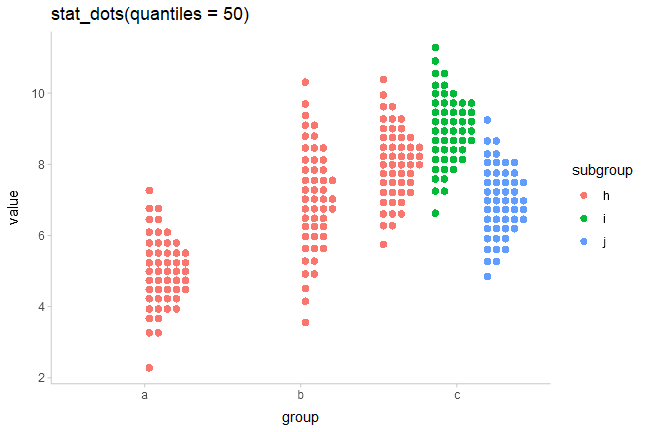For more on dotplots, see `vignette("dotsinterval")`

## Custom plots

The `slabinterval` family of stats and geoms is designed to be very flexible. Most of the shortcut geoms above can be created simply by setting particular combinations of options and aesthetic mappings using the basic `geom_slabinterval()` and `stat_slabinterval()`. Some useful combinations do not have specific shortcut geoms currently, but can be created manually with only a bit of additional effort.

### Gradients of alpha, color, and fill

Two aesthetics of particular use for creating custom geoms are `slab_alpha`, which changes the alpha transparency of the slab portion of the geom, `slab_color`, which changes its outline color, and `fill`, which changes its fill color. All of these aesthetics can be mapped to variables along the length of the geom (that is, the color does not have to be constant over the entire geom), which allows you to create gradients or to highlight meaningful regions of the data (amongst other things). You can also employ the ggdist-specific `color_ramp` and `fill_ramp` aesthetics to create custom gradients with outline and fill colors, as demonstrated later in this section.

Note: The examples of gradients in this section use the (optional) experimental setting `fill_type = "gradient"`. If you do not have R greater than 4.1.0 or are not using a supported graphics device, the output may be blank; in this case, omit this option. Gradients can be produced without this option but they may not look as nice.

By default, `stat_ccdfinterval()` maps the output of the evaluated function (in its case, the CCDF) onto the `thickness` aesthetic of the `slabinterval` geom, which determines how thick the slab is. This is the equivalent of setting `aes(thickness = after_stat(f))`. However, we could instead create a CCDF gradient plot, a sort of mashup of a CCDF barplot and a density gradient plot, by mapping `after_stat(f)` onto the `slab_alpha` aesthetic instead, and setting `thickness` to a constant (1):

``````df %>%
ggplot(aes(x = group, y = value, fill = subgroup)) +
stat_ccdfinterval(aes(slab_alpha = after_stat(f)),
thickness = 1, position = "dodge", fill_type = "gradient"
) +
expand_limits(y = 0) +
# plus coord_cartesian so there is no space between bars and axis
coord_cartesian(expand = FALSE) +
ggtitle("stat_ccdfinterval(thickness = 1)", "aes(slab_alpha = after_stat(f))")``````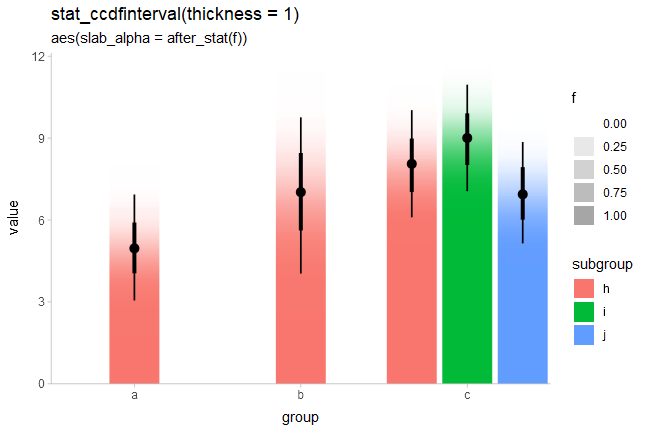If this approach were applied to bins in a histogram, where each bin had some uncertainty associated with its height, the result would be a so-called fuzzygram (Haber and Wilkinson 1982).

### Highlighting and other combinations

The ability to map arbitrary variables onto fill or outline colors within a slab allows you to easily highlight sub-regions of a plot. Taking the earlier example of visualizing priors, we can add a mapping to the `fill` aesthetic to highlight a region of interest, say ±1.5:

``````priors = tribble(
~ dist,      ~ args,
"norm",      list(0, 1),
"student_t", list(3, 0, 1)
)

priors %>%
ggplot(aes(y = dist, xdist = dist, args = args)) +
stat_halfeye(aes(fill = after_stat(abs(x) < 1.5))) +
ggtitle("stat_halfeye()", "aes(fill = after_stat(abs(x) < 1.5)))") +
# we'll use a nicer palette than the default for highlighting:
scale_fill_manual(values = c("gray85", "skyblue"))``````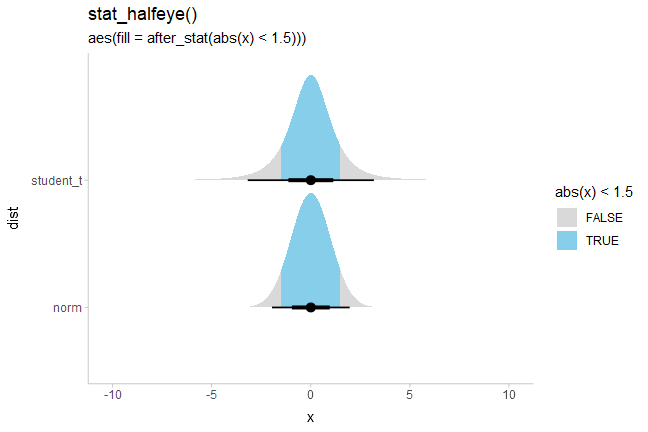We could also combine these aesthetics arbitrarily. Here is a (probably not very useful) eye plot + gradient plot combination, with the portion of the distribution above 1 highlighted:

``````priors %>%
ggplot(aes(y = dist, xdist = dist, args = args)) +
stat_eye(aes(slab_alpha = after_stat(f), fill = after_stat(x > 1)), fill_type = "gradient") +
ggtitle(
"aes(slab_alpha = after_stat(f), fill = after_stat(x > 1)))"
) +
# we'll use a nicer palette than the default for highlighting:
scale_fill_manual(values = c("gray75", "skyblue"))``````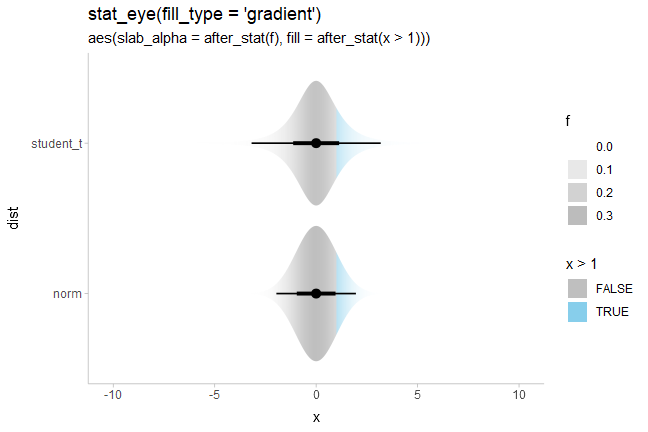### Mashups with Correll and Gleicher-style gradients

We can also take advantage of the fact that all slabinterval stats also supply `cdf` and `pdf` aesthetics to create charts that make use of both the CDF and the PDF in their aesthetic mappings. For example, we could create Correll & Gleicher-style gradient plots by fading the tails outside of the 95% interval in proportion to \(|1 - 2F(x)|\) (where \(F(x)\) is the CDF):

``````priors %>%
ggplot(aes(y = dist, xdist = dist, args = args)) +
stat_gradientinterval(aes(slab_alpha = after_stat(-pmax(abs(1 - 2*cdf), .95))),
) +
scale_slab_alpha_continuous(guide = "none") +
ggtitle(
"aes(slab_alpha = after_stat(-pmax(abs(1 - 2*cdf), .95)))"
)``````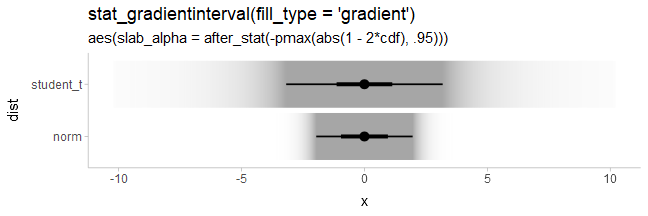We could also do a mashup of faded-tail gradients with violin plots by starting with an eye plot and then using the generated `cdf` aesthetic to fade the tails, producing plots like those in Helske et al.:

``````priors %>%
ggplot(aes(y = dist, xdist = dist, args = args)) +
stat_eye(aes(slab_alpha = after_stat(-pmax(abs(1 - 2*cdf), .95))), fill_type = "gradient") +
scale_slab_alpha_continuous(guide = "none") +
ggtitle(
"aes(slab_alpha = after_stat(-pmax(abs(1 - 2*cdf), .95)))"
)``````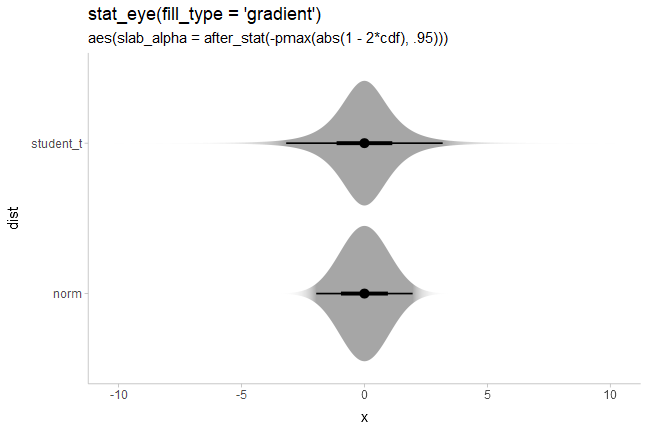A related idea is one from Tukey: rather than visually emphasizing where a value is likely, emphasize where it is unlikely. While Tukey used a visual representation showing both pointwise and simultaneous intervals, for this example we will do something a bit different, inverting the faded-tails function from Correll & Gleicher to create bars that “block out” the regions of low likelihood:

``````dist_df %>%
ggplot(aes(x = group, ydist = dist_normal(mean, sd), fill = subgroup)) +
stat_slab(
aes(
thickness = after_stat(pmax(0, abs(1 - 2*cdf) - .95)),
fill_ramp = after_stat(pmax(0, abs(1 - 2*cdf) - .95))
),
side = "both", position = "dodge", fill_type = "gradient"
) +
labs(
title = 'stat_slab(side = "both")',
subtitle = paste0(
"aes(fill = subgroup,\n       ",
"fill_ramp and thickness = after_stat(pmax(0, abs(1 - 2*cdf) - .95)))"
)
) +
guides(fill_ramp = "none") +
coord_cartesian(expand = FALSE)``````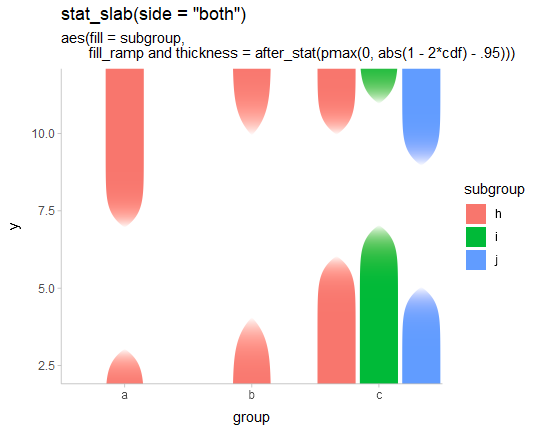Thanks to a tweet from Jessica Hullman that inspired the idea.

### Densities filled according to intervals

Another common chart type involves filling in the interior of a halfeye plot according to some intervals. Here, we can use the fact that computed variables from the interval sub-geometry are made available to the slab sub-geometry and vice versa.

For example, within the slab sub-geometry, the `.width` and `level` computed variables correspond to the smallest intervals that contain the `x` value at that portion of the slab. Thus, we can map `.width` or `level` onto the slab fill:

``````df %>%
ggplot(aes(y = group, x = value)) +
stat_halfeye(aes(fill = after_stat(level))) +
# na.translate = FALSE drops the unnecessary NA from the legend, which covers
# slab values outside the intervals. An alternative would be to use
# na.value = ... to set the color for values outside the intervals.
scale_fill_brewer(na.translate = FALSE) +
labs(
title = "stat_halfeye()",
subtitle = "aes(fill = after_stat(level))",
fill = "interval"
)``````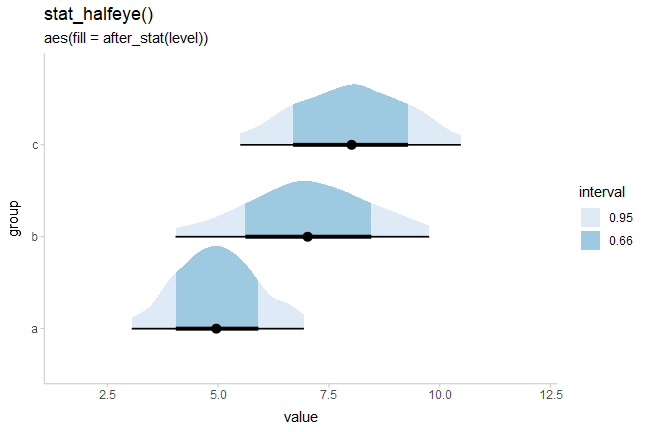(Note: in previous versions of ggdist, using `cut_cdf_qi()` was the recommended way to achieve this affect. That function still exists for backwards compatibility, but mapping `level` or `.width` is now the recommended approach, as it generalizes to other interval types, such as highest-density intervals — see later.)

To apply the color scale to all values outside the intervals, one option is to split `stat_halfeye()` into its constituent parts (`stat_slab()` and `stat_pointinterval()`), then include a “100%” interval in `.width`:

``````df %>%
ggplot(aes(y = group, x = value)) +
stat_slab(aes(fill = after_stat(level)), .width = c(.66, .95, 1)) +
stat_pointinterval() +
scale_fill_brewer() +
labs(
title = "stat_slab()",
subtitle = "aes(fill = after_stat(level), .width = c(.66, .95, 1))",
fill = "interval"
)``````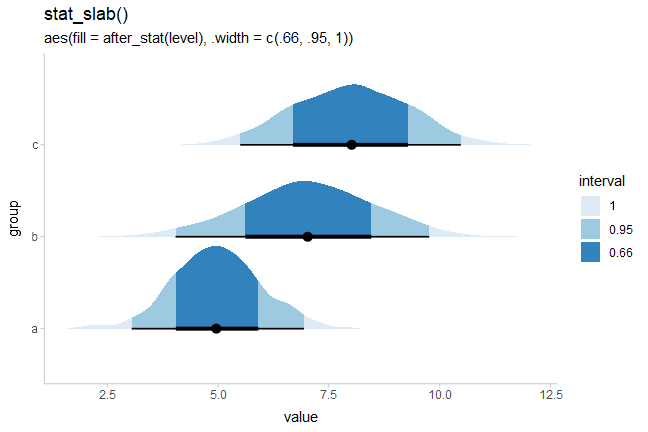If we change the interval type used, the resulting `.width` and `level` computed variables change correspondingly, allowing us to highlight other types of intervals besides quantile intervals; for example, highest-density intervals:

``````qi_plot = data.frame(dist = dist_beta(10, 2)) %>%
ggplot(aes(xdist = dist, fill = after_stat(level))) +
stat_halfeye(point_interval = median_qi, .width = c(.5, .8, .95)) +
scale_fill_brewer(na.value = "gray95") +
labs(subtitle = "stat_halfeye(aes(fill = after_stat(level)), point_interval = median_qi)")

hdi_plot = data.frame(dist = dist_beta(10, 2)) %>%
ggplot(aes(xdist = dist, fill = after_stat(level))) +
stat_halfeye(point_interval = mode_hdci, .width = c(.5, .8, .95)) +
scale_fill_brewer(na.value = "gray95") +
labs(subtitle = "stat_halfeye(aes(fill = after_stat(level)), point_interval = mode_hdci)")

qi_plot /
hdi_plot``````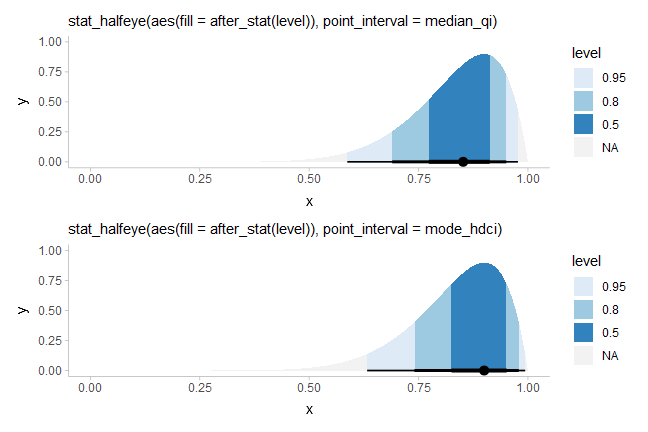### Using color ramps for `fill` and `color` aesthetics

`ggdist` supplies `color_ramp` (or `colour_ramp`) and `fill_ramp` aesthetics which can be used to vary (“ramp”) the outline or fill colors smoothly from a base color (default `"white"`) to whatever color the geometry would otherwise have.

Taking the above example with interval-filled slabs, we could use the `fill_ramp` aesthetic instead of the `fill` aesthetic to set the slab color based on the interval it is in. We could then vary the base fill color separately from the interval based on another column in the original data table, such as the `subgroup` column:

``````df %>%
ggplot(aes(y = group, x = value)) +
stat_halfeye(
aes(fill = subgroup, fill_ramp = after_stat(level)),
.width = c(.50, .80, .95),
# NOTE: we use position = "dodgejust" (a dodge that respects the
# justification of intervals relative to slabs) instead of
# position = "dodge" here because it ensures the topmost slab does
# not extend beyond the plot limits
position = "dodgejust",
) +
# a range from 1 down to 0.2 ensures the fill goes dark to light inside-out
# and doesn't get all the way down to white (0) on the lightest color
scale_fill_ramp_discrete(na.translate = FALSE) +
labs(
title = "stat_halfeye(position = 'dodgejust')",
subtitle = "aes(fill = subgroup, fill_ramp = after_stat(level))",
fill_ramp = "interval"
)``````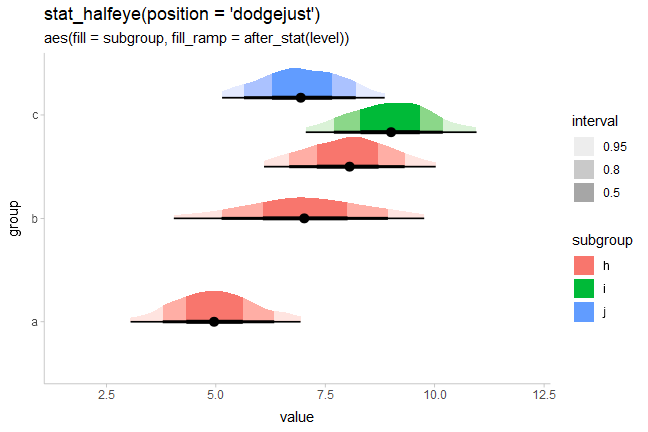We could similarly use `stat_interval()` with the `color_ramp` aesthetic to vary subgroup color separately from the whiteness of the intervals. Here, `level` is a variable generated by all stats in the `stat_slabinterval()` family which contains the level of the generated intervals, as an ordered factor.

``````dist_df %>%
ggplot(aes(x = group, ydist = dist_normal(mean, sd), color = subgroup)) +
stat_interval(aes(color_ramp = after_stat(level)), position = "dodge") +
labs(
title = "stat_interval()",
subtitle = "aes(color = subgroup, color_ramp = after_stat(level))"
)``````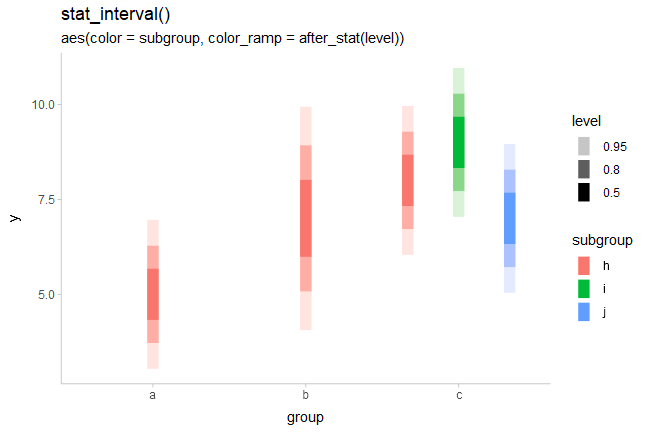See `help("scale_color_ramp")` for more information on the color ramp aesthetics/scales.

### Raindrop plots

Barrowman and Myers proposed an alternative to density-based eye plots (such as created by `stat_eye()` by default) called raindrop plots. In these, the thickness of the slab is proportional to `log(pdf)` instead of `pdf`, and is bounded within the 95% interval. We can construct a function that uses the `pdf` and `.width` computed variables to give a thickness proportional to `log(pdf)` within the 95% interval, and use it to create raindrop plots.

Barrowman and Myers apply this technique with a 95% raindrop superimposed on a 99% raindrop, which we can replicate:

``````priors %>%
ggplot(aes(y = dist, xdist = dist, args = args)) +
# must also use normalize = "groups" because min(log(pdf)) will be different for each dist
stat_slab(
aes(thickness = after_stat(ifelse(.width <= 0.99, log(pdf), NA))),
normalize = "groups", fill = "gray85", .width = .99, side = "both"
) +
stat_eye(
aes(thickness = after_stat(ifelse(.width <= 0.95, log(pdf), NA))),
normalize = "groups"
) +
ggtitle(
'stat_eye(normalize = "groups")',
"with aes(thickness = after_stat(ifelse(.width <= 0.95, log(pdf), NA)))\nand aes(thickness = after_stat(ifelse(.width <= 0.99, log(pdf), NA)))"
)``````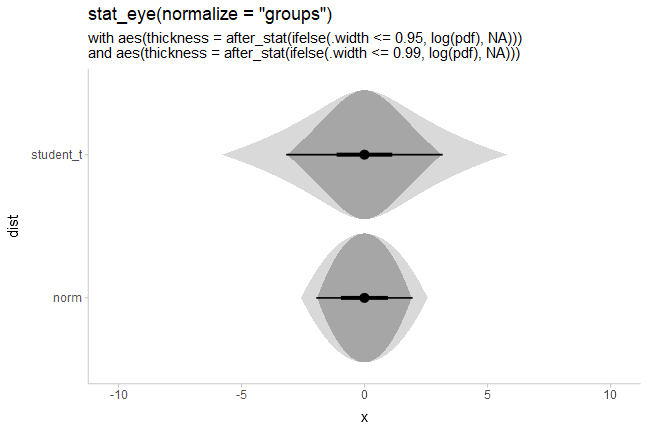### Creating ridge plots

When plotting densities (as in `stat_halfeye()`, `stat_slab()`, etc) it can be useful to overplot many densities simultaneously, an approach sometimes called ridge plots (e.g. as in the ggridges package). This can be done by setting `scale` or `height` to a value greater than 1. Setting `height` is often the best approach as it will correctly adjust plot boundaries (unless you need to use `position = "dodge"`, in which case you should use `scale` and adjust plot boundaries manually).

``````set.seed(1234)

ridges_df = data.frame(
group = letters[7:1],
x = rnorm(700, mean = 1:7, sd = 2)
)

ridges_df %>%
ggplot(aes(y = group, x = x)) +
stat_slab(height = 2, color = "black") +
ggtitle("stat_slab(height = 2, color = 'black')")``````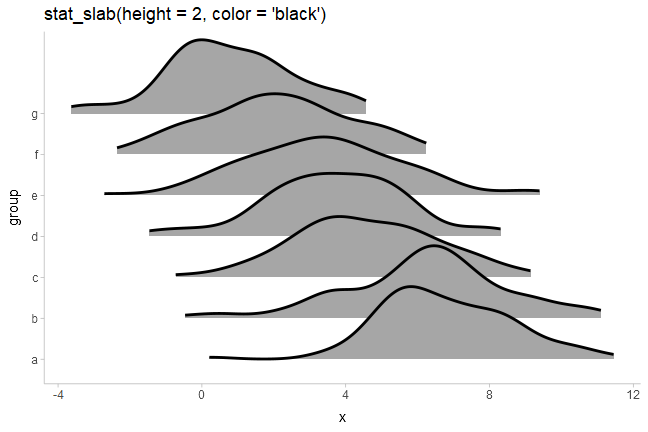Depending on if it makes sense for your data (for example, if the scale is unbounded), you may also wish to adjust the `trim` parameter, which by default restricts the density estimator to 0 outside of the range of the data. Setting `trim` to `FALSE` will ensure the densities smoothly go down to 0. Combining this with `expand = TRUE` will make each slab expand itself to the limits of the `x` axis.

We’ll use `trim` and `expand` along with a combination of `fill` and `fill_ramp` to give each group on the y axis a different color and to vary the fill along the `x` axis in a way that provides a “softer” form of region of practical equivalence:

``````ridges_df %>%
ggplot(aes(
y = group, x = x,
fill = group, fill_ramp = after_stat(abs(x)),
color_ramp = after_stat(-dnorm(x, 0, 2))
)) +
stat_slab(
height = 2, color = "gray15",
expand = TRUE, trim = FALSE,
show.legend = FALSE
) +
geom_vline(xintercept = 0, color = "gray85", linetype = "dashed") +
ggtitle(
'stat_slab(height = 2, color = "black", expand = TRUE, trim = FALSE)',
'aes(fill = group, fill_ramp = after_stat(abs(x)), color_ramp = after_stat(-dnorm(x, 0, 2)))'
) +
scale_fill_viridis_d()``````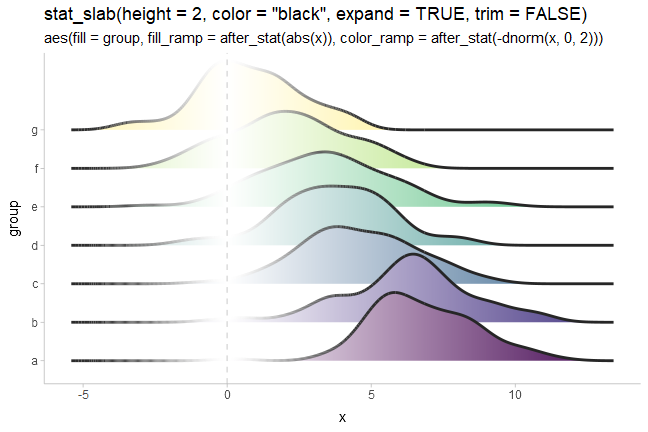We use a tighter ramp on `color` compared to `fill` (via `-dnorm()` instead of `abs()`) because we want the outlines to quickly ramp back to black outside of 0 so that they have sufficient contrast against the slabs when they overlap.

### Varying side, scale, and justification within geoms

The `side`, `scale`, and `justification` parameters can also be varied within all geoms in the `geom_slabinterval()` family, allowing (for example) different groups to hang above or below the interval:

``````dist_df %>%
filter(subgroup == "h") %>%
mutate(side = c("top", "both", "bottom")) %>%
ggplot(aes(y = group, xdist = dist_normal(mean, sd), side = side)) +
stat_dotsinterval(scale = 2/3) +
labs(
title = 'stat_dotsinterval(scale = 2/3)',
subtitle = 'aes(xdist = dist_normal(mean, sd), side = c("top","both","bottom"))'
) +
coord_cartesian()``````